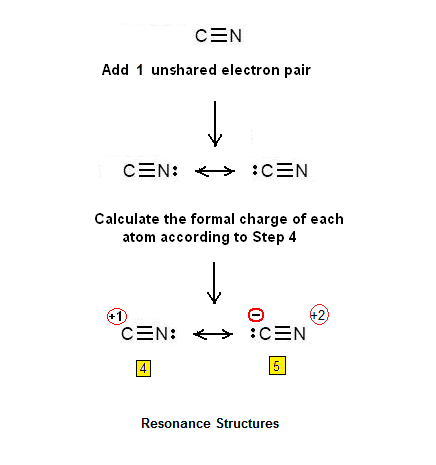Simple Procedure for writing the Lewis Structures of CN+ | Chemistry Net

# Simple Procedure for writing the Lewis Structures of CN+

Simple Procedure for writing Lewis Structures of CN+

# A simple procedure for writing the Lewis structures of CN+

A simple procedure for drawing Lewis dot structures was given in a previous post entitled Lewis Structures and the Octet Rule”.

Several worked examples relevant to this procedure were given in previous posts please see the Sitemap - Table of Contents (Lewis Electron Dot Structures). Let us examine the case Lewis dot structures of CN+.

Let us consider the case of the CN+ cation:

Step 1: Connect the atoms with single bonds.Step 2: Calculate the # of electrons in pi bonds (pi bonds, multiple bonds) using  formula (1)

Where n in this case is 2 since  CN+ consists of 2 atoms.

Where V = (4 + 5 ) - charge  = 9 – 1 = 8

Therefore, P = 6n + 2 – V = 6 *2 + 2 – 8 = 6 Therefore there are 6 pi electrons (pi electrons). So, there are 1) either 3 double bonds or 1 triple bond and an unshared electron pair.

Step 3 & 4: Two atoms cannot be connected with 3 double bonds. However, it is possible that will be connected by a double bond. Two unshared electron pairs will be also added – so that in total 6 pi electrons will be present:Another possibility is that the two atoms will be connected by a triple bond. In this case an unshared electron pair will be added so that 6 pi electrons will be present. The Lewis dot structures of the CN+cation are shown below:Relevant Posts

Lewis Structures and the Octet Rule - A simple method to write Lewis Structures

Lewis Electron Dot Structures of Hydrogen Cyanide (HCN) and Nitronium Ion (NO2+)

Simple method for writing Lewis Structures – Ozone O3 and carbonate CO3-2

Simple Method for drawing Lewis structures (Video): H2CO3

References

1. G.N. Lewis, J.A.C.S, 38, 762-785, (1916)
2.  E. C. McGoran, J. Chem. Educ., 68, 19-23 (1991)
3. A.B.P. Lever, J. Chem. Educ., 49, 819-821, (1972)

Key Terms

electron dot Lewis structures, Lewis Structures and the Octet Rule, how can we draw the CN+ Lewis dot structure